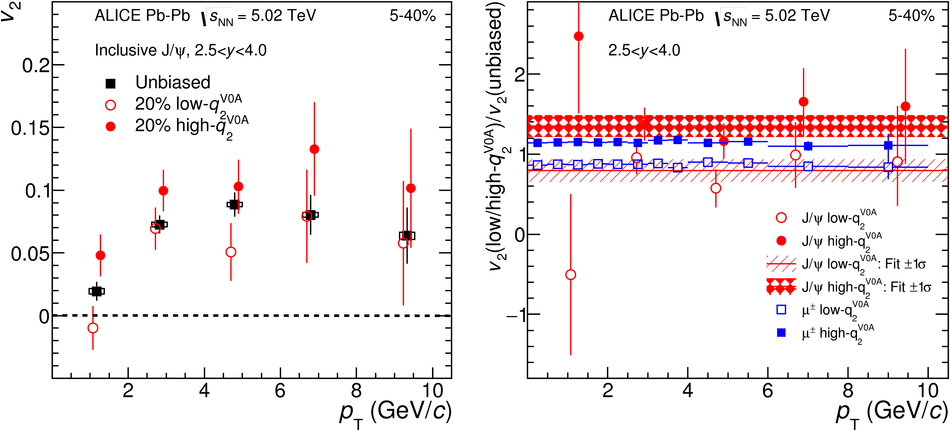# Figure 7

 Left: The \jpsi\ $v_2$ as a function of $p_{\rm T}$ for shape selected and unbiased samples in the 5--40\ Pb--Pb collisions at $\sqrt{s_{\rm NN}}$ = 5.02 TeV. Points are slightly shifted along the horizontal axis for better visibility. Statistical and systematic uncertainties are shown as bars and boxes, respectively. Right: Ratio of the \jpsi\ $v_2$ in lowest and highest $q_2^{\rm V0A}$ event-shape classes and the unbiased sample The shaded bands represent the result with a constant function $\pm$1$\sigma$ The \jpsi\ results are compared to the ratios for the single muons $v_2$ obtained with the same event-shape classes.